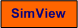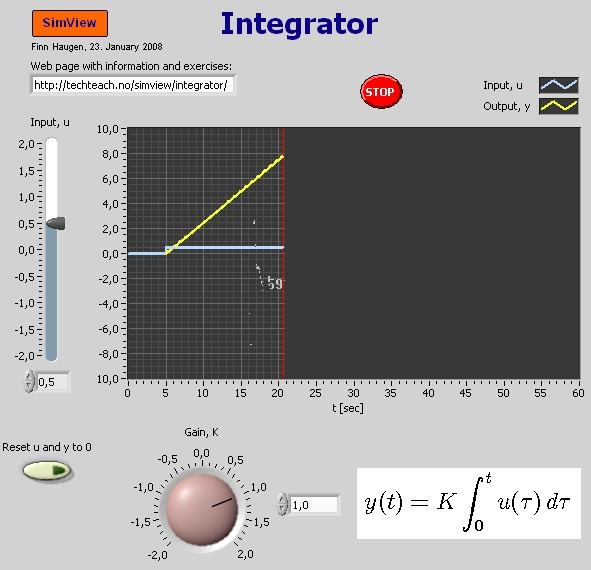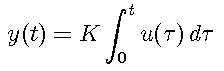# Integrator

Snapshot of the front panel of the simulator:• What is needed to run the the simulator? Read to get most recent information!
• Tips for using the simulator.
• The simulator: . The simulator runs immediately after the download by clicking Open in the download window. Alternatively, you can first save a copy of the exe-file on any directory (folder) on your PC and then run the exe-file, which starts the simulator.

## Description of the simulated system

In this simulator a general integrator is simulated. You can freely adjust the input signal u(t) and the gain of the integrator, K.

The relation between the input signal u and the output signal y can be expressed with the following integral equation:This integral equation is equivalent with the following differential equation:

dy(t)/dt = Ku(t)

The transfer function of the integrator is

h(s) = y(s)/u(s) = K/s

The simulator is based on discretizing the integrator with the Runge-Kutta second-order method with time-step of 0.05 sec.

## Aims

The aims of the simulator is to give insight into the dynamic behaviour of an integrator.

## Motivation

There are many dynamic systems with interating behaviour, e.g.:

• Liquid tanks where the outflow is level is independent of the level, as if the outflow is produced by a pump. In such a system the pump control signal can be the input variable and the level is the output variable of the integrator.
• Thermal systems without heat loss to the environment. The iInput variable is heat supply (via a heatring element), and the output variable is the temperature.
• A motor having neglectable (or very quick) dynamics. The input variable is the motor control signal, and the output variable is the motor rotational position.
• The integrator-term of a PID-controller. The input variable is the control error, and the output variable is the integrator term, ui.

Below, U is the amplitude of the input step, and K is the gain of the integrator.

1. The shape of the step response: Set K = 1. Simulate with an input step amplitude of U = 1. Characterize the shape of the step response.

2. The importance of the gain K: Simulate with input step amplitude U = 1 for different values of K, both positive and negative. How does the slope of the step response depend on K?

3. The integrator effect: Simulate while adjusting the input u(t). Do you see that the integrator actually integrates ("stores") the input?

[SimView] [TechTeach]

Updated 23. January 2008. Developed by Finn Haugen. E-mail: finn@techteach.no.